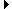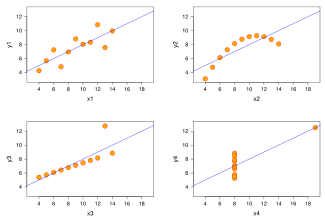Members AreaSign Up | Log In
 Free Level 2 QuotesStock AnalysisCorrelation Trading PlatformCorrelationsMembership Benefits

The stock analysis page contains stock tools allowing you to find correlations between stocks.

Linear correlation can reveal stocks that have moved up or down in percentage terms either together or opposite of each other. AiStockCharts.com uses the median % daily price change of 2 stocks in the calculation of the linear correlation coefficient. This differs slightly from many websites that use the mean instead of the median. All of the data is still considered. An equal number of outliers at either extreme of the data do not influence the median (the value in the middle). However, the mean (average calculated by adding up all the data and dividing by the number of data points) can be greatly influenced by outliers, especially for small sample sizes. An example of this phenomenon is a stock specific event where the stock shoots up 185% in 1 day. Stocks can have a non-symmetrical distribution of % daily gains. Stocks can lose 100% of their value, however can gain in price by a much larger percentage.

Scatter plots help identify the nature of the distribution of daily % stock price changes. The problem in relying solely on a correlation coefficient to describe the strength of the relationship between data sets is shown on the scatter plots below. Scatter plots that consist of all data points forming either a vertical or horiztonal line indicate that the linear corrleation is undefined. Linear correlation exists between the 2 variables only if each variable has a non-zero finite standard deviation.Anscombe Quartet constructed in 1973 by Frank Anscombe

All 4 response variables have the same mean (7.5), standard deviation (4.12), correlation (0.81) and regression line (y = 3 + 0.5x). However, as can be seen on the plots, the distribution of the variables is very different. The first one (top left) seems to be distributed normally, and corresponds to what one would expect when considering two variables correlated and following the assumption of normality. The second one (top right) is not distributed normally; while an obvious relationship between the two variables can be observed, it is not linear, and the Pearson correlation coefficient is not relevant. In the third case (bottom left), the linear relationship is perfect, except for one outlier which exerts enough influence to lower the correlation coefficient from 1 to 0.81. Finally, the fourth example (bottom right) shows another example when one outlier is enough to produce a high correlation coefficient, even though the relationship between the two variables is not linear. These examples indicate that the correlation coefficient, as a summary statistic, cannot replace the individual examination of the data.

Linear correlation coefficient values range between -1 and 1. It is important to understand that high correlation does not necessarily mean that there is an underlying fundamental relationship between the stocks, however can offer some insight for further investigation. A correlation value of 0 suggests that the stocks daily percentage movements were independent of each other (not correlated). We cannot state that historical data causes a specific move in a stock since new variables can crop up anytime and make the model invalid or unpredictable. Also, there is the possibility of pure coincidence or confounding variables creating the appearance of a relationship between 2 stocks when in fact there is none.

AiStockCharts.com plots a best-fit line on the scatter plot of 2 stocks to show the relationship of the variation between the 2 stocks. This relationship is quantified by BETA and shown on the graph using �, the slope of the best-fit line. BETA is commonly used in finance and often calculated using ordinary least squares regression. When calculated this way the BETA is the slope of the fitted line resulting from the least squares regression calculation. The problem with the usual interpretation is that BETA when calculated this way is not comparing the relative volatility (variation between the 2 stocks). Instead, it is the correlation coefficient multiplied by the relative volatility. Therefore, a low beta could represent a high relative volatility masked by a low correlation. Investors would then be mistaken in thinking that they had selected an investment with low relative volatility.

To avoid this common mis-interpretation AiStockCharts uses the ratio of the variation (standard deviations) of the 2 stocks daily % changes multiplied by the sign of the correlation coefficient. Tofallis, Chris, 'Investment Volatility: A Critique of Standard Beta Estimation and a Simple Way Forward' . University of Hertfordshire Business School Working Paper No. 2004:3 provides a discussion of this, together with a real example involving AT&T. The graph showing monthly returns from AT&T is visibly more volatile than the index and yet the standard estimate of beta for this is less than one.

The BETA calculation affects the value of ALPHA, a commonly used metric to compare investments or investment managers performance. ALPHA is the y-intercept of the best-fit line (where the purple line crosses the vertical yellow y-axis). ALPHA represents the estimated extra % profit (when positive) generated by the stock plotted on the y-axis versus the stock on the x-axis when the percent change of the stock on the x-axis is zero. ALPHA is shown at the top of the scatter plot to the left of �.

Two huge problems can result from the common way of calculating � in finance. First, the absolute value of � is too small. Second, ALPHA can be overestimated or underestimated depending on the location of the centroid represented by the intersection of the median daily % price changes for the stock and the index. In other words, risk is underestimated and reward can be overestimated or underestimated. As mentioned above, AiStockCharts does not use the common way of calculating �. The following table shows problems that can arise when using the standard estimate for �.

 Sign of CC * Quadrant of medians Implications on green ticker when using the standard estimate of � - II Reward overestimated, Risk underestimated - III Reward overestimated, Risk underestimated + I Reward overestimated, Risk underestimated + IV Reward overestimated, Risk underestimated - I Reward underestimated, Risk underestimated - IV Reward underestimated, Risk underestimated + II Reward underestimated, Risk underestimated + III Reward underestimated, Risk underestimated

* Based on the Cartesian coordinate system.
Assumes a linear relationship exists and the magnitude of CC is sufficiently large to make estimates.
AiStockCharts does not use the standard estimate for �.

r� (the correlation coefficient squared) is a measure of how predictable the least squares regression line was in the past to predict the daily % change of the stock on the y-axis when given a daily % change in stock price for the stock on the x-axis. Aistockcharts.com uses r� based on my modified correlation coefficient calculation in order to calculate RR (Adjusted Reward to Risk Ratio). The correlation coefficient (CC on the scatter plot) is an indicator showing how closely correlated the stocks daily % changes in price are to each other. Outliers are excluded.